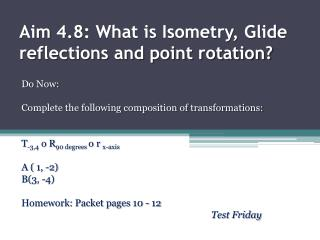Download PresentationAim 4.8: What is Isometry, Glide reflections and point rotation?

# Aim 4.8: What is Isometry, Glide reflections and point rotation? - PowerPoint PPT Presentation

Aim 4.8: What is Isometry, Glide reflections and point rotation?. Do Now: Complete the following composition of transformations: T -3,4 o R 90 degrees o r x-axis A ( 1, -2) B(3, -4) Homework : Packet pages 10 - 12 Test Friday.I am the owner, or an agent authorized to act on behalf of the owner, of the copyrighted work described.
Download Presentation## Aim 4.8: What is Isometry, Glide reflections and point rotation?

Download Policy: Content on the Website is provided to you AS IS for your information and personal use and may not be sold / licensed / shared on other websites without getting consent from its author.While downloading, if for some reason you are not able to download a presentation, the publisher may have deleted the file from their server.

- - - - - - - - - - - - - - - - - - - - - - - - - - E N D - - - - - - - - - - - - - - - - - - - - - - - - - -
Presentation Transcript
1. Aim 4.8: What is Isometry, Glide reflections and point rotation? Do Now: Complete the following composition of transformations: T-3,4 o R90 degrees o r x-axis A ( 1, -2) B(3, -4) Homework: Packet pages 10 - 12 Test Friday

2. Aim 4.8: What is Isometry, Glide reflections and point rotation? Homework Answers: Packet p. 1 • a) (2,4)  (5, -1)  (-5, -1) b) (-3, -4)  (4, -3)  (8, -6) Packet p. 2 • b) A’ (-2, -2) B’ (-7, -4) C’ (-5, -6) c) A’’ (1, -6) B’’ (-4, -8) C’’ (-2, -10) • b) A’ (1, 3) B’ (3, 4) C’ (1, 6) c) A’’ (-3, 1) B’’ (-4, 3) C’’ (-6, 1) d) A’’’ (3, -1) B’’’ (4, -3) C’’’ (6, -1)

3. Aim 4.8: What is Isometry, Glide reflections and point rotation? What is an Isometry? • Any transformation that preserves distance is a isometry • The only transformation that does NOT preserve isometry: Dilations • A direct isometry: any isometry that also preserves orientation. • Translations & Dilations • An opposite isometry reverses orientation • Reflections

4. Aim 4.8: What is Isometry, Glide reflections and point rotation? What is a Glide Reflection? • Glide reflection: A translation and a reflection in the same composition. • The line of reflection is parallel to the translation. • Glide reflections are always compositions. • Everything except orientation is preserved in a Glide reflection

5. Aim 4.8: What is Isometry, Glide reflections and point rotation? Examine the graph.  Is triangle A"B"C" a glide reflection of triangle ABC? Yes!

6. Aim 4.8: What is Isometry, Glide reflections and point rotation? Perform the following composition of transformations. Is this a Glide reflection? T -5, 0 o r y=2 U (2, 0) S (6, 0) A (4, -3)

7. Aim 4.8: What is Isometry, Glide reflections and point rotation? Perform the following composition of transformations. Is this a Glide reflection? r y=x o T -2, -2 A (3, 1) B (8, 1) C (5, 3)

8. Aim 4.8: What is Isometry, Glide reflections and point rotation? Work: r y=x o T -2, -2 A (3, 1) A’ (1, -1) A’’ (-1, 1) B (8, 1) B’ (6, -1) B’’ (-1, 6) C (5, 7) C (3, 5) C’’ (5, 3) T -2, -2 r y=x r y=x T -2, -2 r y=x T -2, -2

9. Aim 4.8: What is Isometry, Glide reflections and point rotation? Point Rotations • A point rotation rotates a figure around one of it’s vertexes • Each turn of the figures represents a certain number of degrees • Formula: 360o / Number of sides • Examples:

10. Aim 4.8: What is Isometry, Glide reflections and point rotation? Example: In the figure below, what point will A be mapped to after the transformation R 120o? B A C F E D

11. Aim 4.8: What is Isometry, Glide reflections and point rotation? Point Reflections: • We want to visualize folding our picture over the line of symmetry and finding our new set of points. • The point or line that is opposite our original side or angle, is our result after the transformations. A B D C

12. Aim 4.8: What is Isometry, Glide reflections and point rotation? Example: n R90 degrees o r n ( A ) A B B C D

13. Aim 4.8: What is Isometry, Glide reflections and point rotation? Exit Ticket: • Create an example of composition which is also a glide reflection. • Use this glide reflection on the point P (-2, 3) to determine point P’’